Latest Teaching jobs   »   Properties Of Circle Part 2 :...

# Properties Of Circle Part 2 : Download Mathematics Study Notes Free PDF For REET Exam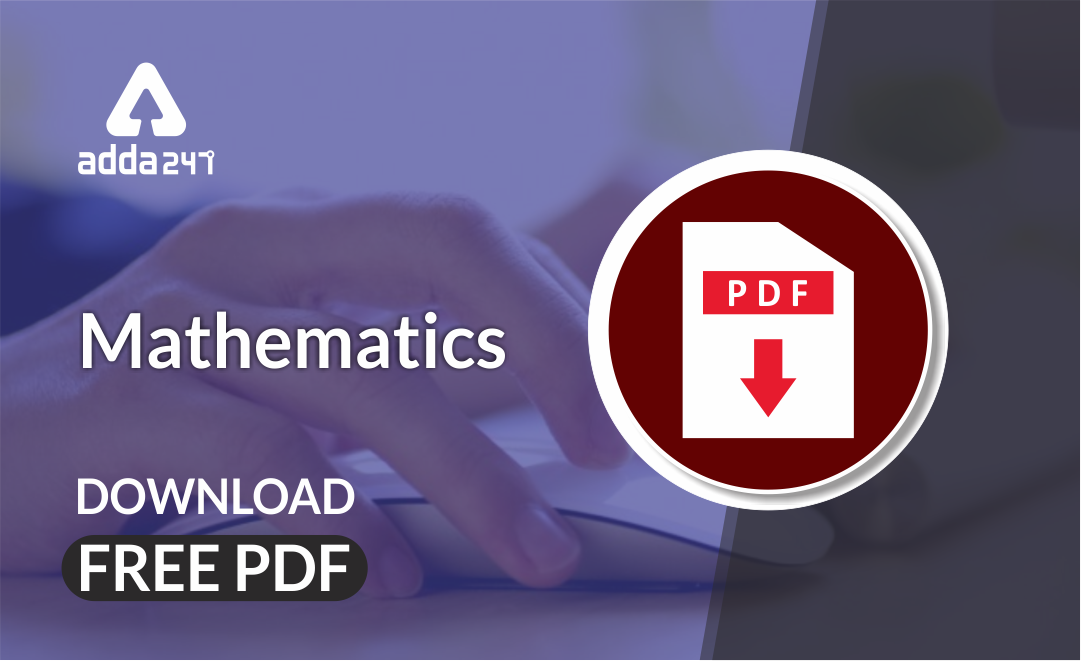Mathematics is an equally important section for  REETMPTET & DSSSB Exams and has even more abundant importance in some other exams conducted by central or state govt. Generally, there are questions asked related to basic concepts and Facts of the Properties of the circles.

To let you make the most of Mathematics section, we are providing important facts related to the Properties of the circles. At least 2-3 questions are asked from this topic in most of the teaching exams. We wish you all the best of luck to come over the fear of the Mathematics section.

How to Overcome Exam Fever, Especially When You Fear Maths

## Properties of Circle Part 2

• If a side of a cyclic quadrilateral is produced then the exterior angle is equal to the interior opposite angle.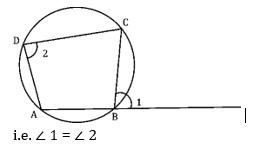• If two chords AB and CD interest internally or externally at a point, E, Then, AE × EB = CE × ED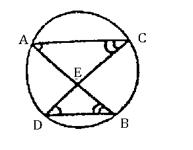• If a secant and a tangent externally interested each other.

then, PT² × PB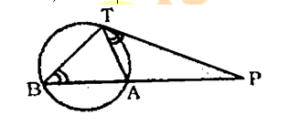• AE is angle bisector of ∠ BAC then AB . AC + DE . AE = AE²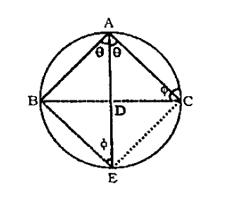Get free Study material for REET Exam

• If two chords AB and AC of a circle are equal, then the bisector of ∠ BAC passes through the center O of the circle. ∠ 1 = ∠ 2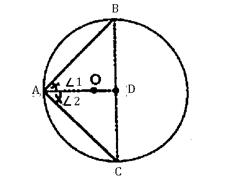Tangent: – A line meeting a circle in only one point is called a tangent.

1. A tangent at any point of a circle is perpendicular to the radius through the point of contact.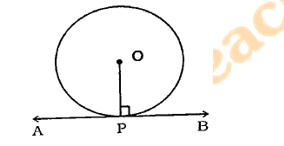i.e. If AB is a tangent at P, then OP  AB.

Practice REET Previous Year Maths Quiz For REET Exam Here

1. The lengths of two tangent, drawn from and external point to a circle, are equal. i.e. AP = AQ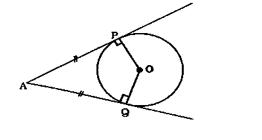1. The angle between a chord and a tangent drawn at end point of chord is equal respectively to the angle formed in the corresponding alternate segments.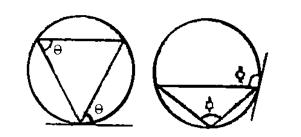Mathematics Study Notes For All Teaching Exams

1. If two circles touch each other internally or externally the point of contact lies on the line joining their centres.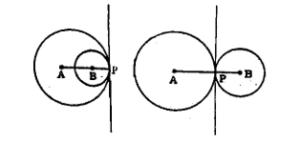i.e. A, B and P are collinear.

Distance between their centres (d)

• When touch internally, d = AP – BP
• When touch externally, d = AP + BP
1. Two circles externally touch each other at P. AB is direct common tangent (DCT) of the circles. If ∠ BAP = x then ∠ ABP = 90° – x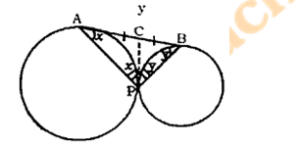Remember, ∠ APB is always right angle .

•विशेषण- परिभाष�...
•Phases of Teaching - Stages of Teaching ...
•Operant and Classical Conditioning - Dif...
•संज्ञा: परिभाष�...
•अलंकार - परिभाष�...
•Stages of Development- Child Growth and ...